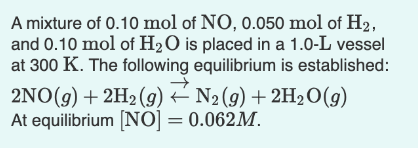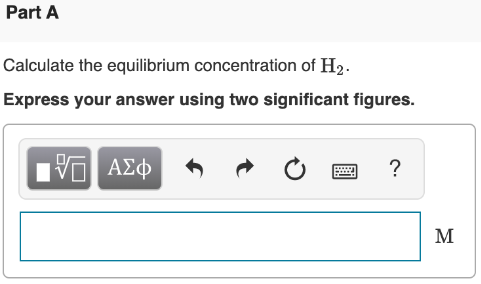# A mixture of 0.10 mol of NO, 0.050 mol H2, and 0.10 mol of H2O is placed in a 1.0-L vessel at 300 K. The following equilibrium is established: 2NO(g) + 2H2(g) ⇌ N2(g) + 2H2O(g) At equilibrium [NO] = 0.062M. Calculate the equilibrium concentration of H2. Express your answer using two significant figures.